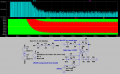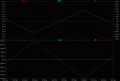# Coupling capacitor for DC filtering

#### alpheratz

Joined Nov 10, 2019
17
I try to eliminate DC component of an input triangular wave. Average value 7.5V and peak to peak value 5V (so from 5 to 10V). Frequency varies from 200Hz to 40 kHhz. I also want to amplify it (about 3x but anyway).
I use an op amp (opa277).
What should I worry about with this configuration? (see figure).
I think even for low frequency it should work, common mode input resistance of my op amp is something like 250 GOhm, therefore even at 200Hz (1/sC= 800kOhm) I shouldn't have attenuation in the forming voltage divider. The other components are all odd multiplies of 200Hz so I don't have anything below this frequency right? I'm afraid of loosing amplitude because of that, or to ruin the quality of my waveform.
Unfortunately my simulator suffers because of that long needed time constants till it gets alternating and so its computationally difficult for me to check it. I don't have any way to check it practically (still closed laboratory because of covid). I hope your theoretical knowledge would help me.

Last edited:

#### BobaMosfet

Joined Jul 1, 2009
1,898
Your schematic doesn't define your resistor values, and your Capacitor is on your reference input, not on your inverting input, which suggests to me you are uncertain about how OpAmps operate. You might be better off using a BJT for simple amplification.

•alpheratz

#### alpheratz

Joined Nov 10, 2019
17
R11=10kOhm=R12. It operates as non inverting amplifier with gain equal 2.

#### ci139

Joined Jul 11, 2016
1,696
eliminate DC component of an input
one way (slow -- at least before settling) is to integrate (or detect the HIGH/LOW peaks and average) the output and extract it from the input

Last edited:
•alpheratz

#### Hymie

Joined Mar 30, 2018
1,003
Apart from BobaMosfet’s observations on your circuit, when using a capacitor to pass an a.c. signal, the impedance of the capacitor is given by the equation:-

Z = 1/(2.π.f.C)

Where:-

f is the frequency in Hertz

C is the capacitance in Farads

As an example, if we have a frequency of 200Hz and a capacitance of 1µF, then the impedance of the capacitor becomes:-

Z = 1/(2xπx200x1x1.e-6) = 796Ω

You will observe from the above equation that as the frequency increases, the a.c. capacitive impedance decreases.

•alpheratz

#### alpheratz

Joined Nov 10, 2019
17
Z = 1/(2xπx200x1x1.e-6) = 796Ω
yes I did the same calculation but I did a big mistake (I put e^(-9) I think in the formula)
Thanks for the correction.

Thanks Ci139 for this solution. It's sounds a bit complex though. I think I already heard about this approach. This is usually done for fast op amps to eliminate their dc offset voltage where precision is also required. So they use as integrator a slower op amp but with very small dc offset (this is possible to find) as "dc offset corrector" of the faster one...

So my idea is bad and wouldn't work?

Last edited:

#### ci139

Joined Jul 11, 2016
1,696
idea wouldn't work?
everything works somehow for some purpose ... depending on speciffic op amp your circuit may work , but generally you use a resistor from non-inverting input to GND for DC path . . . http://tinyurl.com/y8awqyro (Falstad is a poor at realistic simulations but give some idea)
LTSpice v.Last edited:
•alpheratz

#### crutschow

Joined Mar 14, 2008
28,204
Basic op amp theory.
You cannot connect only a capacitor to an opamp input, as there's no place for the input bias current to go.
There has to be a resistor from the input to ground (or some reference voltage).
Its maximum resistor value depends upon the input bias current of the selected opamp and how much offset voltage from this current is tolerable.

•Tako and BobaMosfet